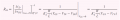# MOS On-resistance, small signal output resistance

#### vqtuyen

Joined Oct 1, 2015
5
1. The resistance of the MOS in triode region near the origin (deep triode), called the on-resistance Ron, is definedRon is also equal to the ratio Vds/Ids
Reference: Microelectronics Circuit Design 4edition - page 154
Firstly, in the triode region, when Vgs increases, channel charge density also increases, and this will reduce the resistance of the channel for sure, and we get the on-resistance Vgs relationship above, but how about when the mos in saturation, the channel length depends on vds (channel length modulation), i think this will change the on resistance.
So the question is how about the on resistance if the NMOS operating in the saturation region? Can we use the above formula for saturation or what formula?
2. I am confusing on resistance of NMOS with small signal output resistance.
Based on the formula ro = 1/(lamda.id). the channel length modulation make the output resistance smaller rather than infinity.
what is the physics behind that causes small signal output resistance?

Thanks very much!

#### Bordodynov

Joined May 20, 2015
2,600
Actually it does not channel modulation and channel length modulation. Due to the reduction of the channel length "L" current increases. It follows that Lambda~1/L. And Ro~L (proportional). There is an expansion volumetric region of the p-n junction of the transistor drain. This property is a semiconductor pn junction: to expand with increasing voltage.

Last edited:

#### dannyf

Joined Sep 13, 2015
2,197
Apples and oranges. Rdson is a useful concept in the linear region for digital applications.

for linear applications, it has no meaning.

#### Bordodynov

Joined May 20, 2015
2,600
I used the MOS transistor as a variable resistor for adjusting the amplification.
I agree that we are talking about very different modes of use of the transistor.
In the first case, the small drain voltage of the transistor. In the second case, at big voltage at the drain of the transistor (amplifying mode).

#### vqtuyen

Joined Oct 1, 2015
5
If there is no channel length modulation, how can you explain about the infinite resistance of the channel physically?

#### Bordodynov

Joined May 20, 2015
2,600
Ro_ac=d_Uds/d_Id (when Lambda=0 d_Id/d_Uds=0 and Ro_ac=1/0), Ro_dc= Uds/Id<infinite.

#### crutschow

Joined Mar 14, 2008
24,953
When the MOSFET is operating as a switch in the linear region, then the Rds is of interest.
When the MOSFET is used as an amplifier it is normally in the saturation region where it is acting as a voltage-current converter with very high Rds .